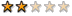## [教學]PhpBB - BBCode研究

shihchieh### [教學]PhpBB - BBCode研究

BBCode研究入門

lang_main.php <--> posting.php <--> posting_body.tpl
bbcode.php <--> bbcode_body.tpl

1.首先在語系檔lang_main.php內新增 bbcode功能說明
\$lang['bbcode_b_help'] = '粗體: [b]text[/b] (alt+b)';

2.接著來到 posting.php 將語系新增的變數 指定給html要用的變數

//
// Output the data to the template
//
\$template->assign_vars(array(

ok，回到前面，再後面加入\r
'L_BBCODE_B_HELP' => \$lang['bbcode_b_help'],

3.接著對應到posting_body.tpl

b_help = "{L_BBCODE_B_HELP}";

4.再下來看到 // Define the bbCode tags 這裡，這裡是設定bbcode功能鈕的基本資料
bbcode = new Array();

bbtags = new Array('[b]','[/b]','[i]','[/i]','[u]','[/u]','[quote]','[/quote]','[code]','[/code]','[list]','[/list]','[list=]','[/list]','[img]','[/img]','[url]','[/url]','[fade]','[/fade]','[scroll]','[/scroll]','[flash width=100 height=100]','[/flash]','[flipv]','[/flipv]','[fliph]','[/fliph]','[stream]','[/stream]','[left]','[/left]','[right]','[/right]','[php]','[/php]','[hide]','[/hide]','[iframe width=500 height=420]','[/iframe]','[rainbow]','[/rainbow]','[real]','[/real]','[blur]','[/blur]','[qt]','[/qt]','[strike]','[/strike]','[wave]','[/wave]','[mod]','[/mod]');

imageTag = false;

5.接下來是顯示的部份要了解的話你必須仔細研究並且了解html的框架結構，這段比較長，請用心看吧~.~"
(tr代表垂直分割，就像一行一行的樣子，而td代表橫向分割，就像一列一列，所按鈕要由左至右排當然是用td，而換行就是用tr囉)

BBCODE按鈕的顯示區塊由此啟始
<td class="row2" valign="top">
<table width="450" border="0" cellspacing="0" cellpadding="2">

<tr align="center">

<td>
<input type="button" class="button" accesskey="b" name="addbbcode0" value="粗體" style="font-weight:bold; width: 52px" onclick="bbstyle(0)" onmouseover="helpline('b')" />

</td>

<td>
<input type="button" class="button" accesskey="i" name="addbbcode2" value="斜體" style="font-style:italic; width: 52px" onclick="bbstyle(2)" onmouseover="helpline('i')" />

</td>

</tr>

<tr align="center">
<td>
<input type="button" class="button" accesskey="e" name="addbbcode18" value="褪色" style="width: 52px" onclick="bbstyle(18)" onmouseover="helpline('e')" />
</td>
</tr>

<td colspan="9">

<table width="100%" border="0" cellspacing="0" cellpadding="0">

<tr>
<td nowrap="nowrap" class="genmed">

{L_FONT_SIZE}:

<option value="{T_BODY_TEXT}">{L_COLOR_DEFAULT}</option>
<option value="7">{L_FONT_TINY}</option>

</select>

{L_ALIGN}:
<option value="{T_BODY_TEXT}">{L_COLOR_DEFAULT}</option>
</select>

</td>
<td nowrap="nowrap" align="right" class="genmed"><a href="javascript:bbstyle(-1)" onmouseover="helpline('a')">{L_BBCODE_CLOSE_TAGS}</a> </td>

</tr>

<tr>
<td nowrap="nowrap" class="genmed">
{L_GLOW_COLOR}:
<option style="color:black; background-color: {T_TD_COLOR1}" value="{T_FONTCOLOR1}" class="genmed">{L_COLOR_DEFAULT}</option>
</select>
</td>
</tr>

</table>

</td>
</tr>

<SCRIPT language=JavaScript src="templates/fisubsilversh/color.js"></SCRIPT>

<TR>
<TD colSpan=12>

<TABLE id=ColorPanel cellSpacing=0 cellPadding=0 align=left
border=0>
<TBODY>
</TBODY>
</TABLE>

</TD>
</TR>
<tr>
<td colspan="9">

<input type="text" name="helpbox" size="45" maxlength="100" style="width:450px; font-size:10px" class="helpline" value="{L_STYLES_TIP}" />
</td>
</tr>

<tr>
<td colspan="9">

<textarea name="message" rows="15" cols="35" style="width:450px" tabindex="3" class="post" onselect="storeCaret(this);" onclick="storeCaret(this);" onkeyup="storeCaret(this);">{MESSAGE}</textarea>
</td>
</tr>

</table>
</td>
BBCODE按鈕的顯示區塊由此結束

1.首先看到bbcode.php

2.裡面就是被posting呼叫後判斷文章內有沒有使用bbcode然後將[x] 包含此區段的內容 [/x]這段作處理

function prepare_bbcode_template(\$bbcode_tpl)

*function bbencode_second_pass(\$text, \$uid)
// [b] and [/b] for bolding text.
\$text = str_replace("[b:\$uid]", \$bbcode_tpl['b_open'], \$text);
\$text = str_replace("[/b:\$uid]", \$bbcode_tpl['b_close'], \$text);
*function bbencode_first_pass(\$text, \$uid)
// [b] and [/b] for bolding text.
\$text = preg_replace("#\[b\](.*?)\[/b\]#si", "[b:\$uid]\\\1[/b:\$uid]", \$text);

3.再來看到bbcode.tpl

<!-- BEGIN b_open --><span style="font-weight:bold"><!-- END b_open -->
<!-- BEGIN b_close --></span><!-- END b_close -->

<span style="font-weight:bold"> text </span>的樣式來顯示，而該文章的內容不變，只是顯示的方式改變

---
Do you fear death ?